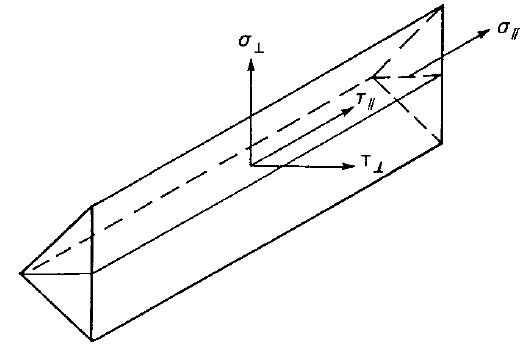# Check of welds according to Chinese standard

$$Fillet welds are checked according to GB 50017 - Chapter 11. The strength of butt welds is assumed the same as the base metal and is not checked.Butt weldsFull penetration butt welds are expected and their resistance is considered equal to that of the parent metal – Cl. 11.2.1.Fillet weldsDesign resistance of fillet welds is checked according to Cl. 11.2.2.2:$\sigma_w = \sqrt{ \left ( \frac{\sigma_f}{\beta_f} \right ) ^2 + \tau_f^2} \le f_f^w$where: σf – stress on the effective area of the weld perpendicular to the weld length βf – enlargement coefficient for design value of fillet weld strength; βf = 1.22 for static loading and angle between fusion faces α = 90°; otherwise βf = 1.0 τf – shear stress on the effective area of the weld parallel to the weld length ffw – design fillet weld strength Design fillet weld strength ffw for welding electrodes; derived from Table 4.4.5 Electrode$$f_f^w$$ [MPa] E43160 E50200 E55220 E60240 Default electrodes are E43 for weakest connected plate with fu < 470 MPa, E50 for 470 MPa ≤ fu < 520 MPa, and E55 for 520 MPa ≤ fu.The weld diagrams show stress according to the following formula:$\sigma = \sqrt{ \frac{1}{\beta_f^2}(\sigma_{\perp}^2 + \tau_{\perp}^2) + \tau_{\parallel}^2 }$$$